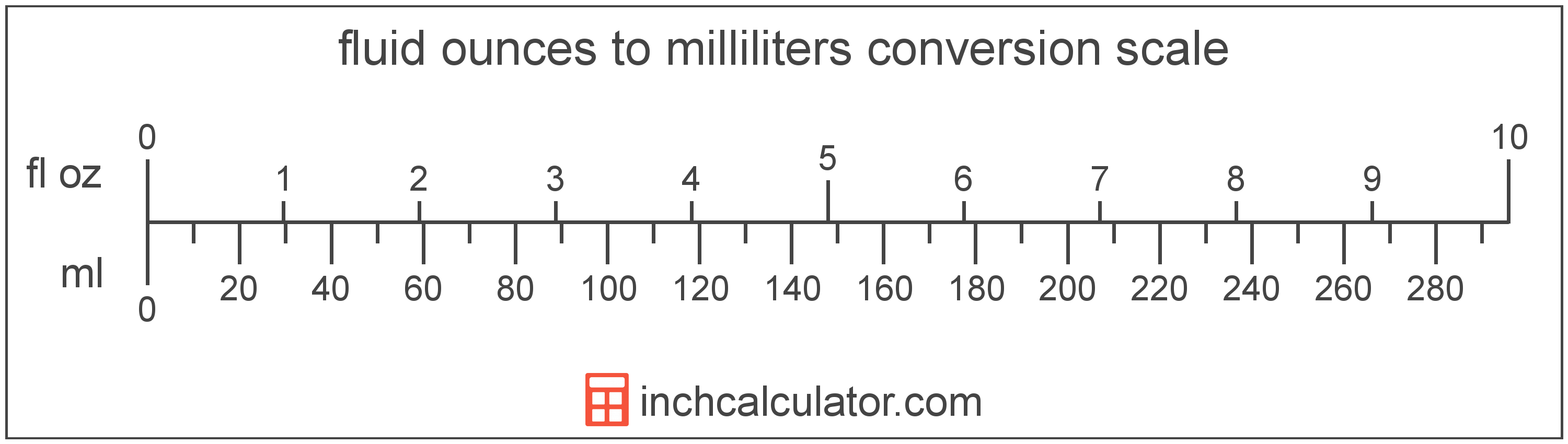# Milliliters to Fluid Ounces Converter

Enter the volume in milliliters below to get the value converted to fluid ounces.

Results in Fluid Ounces:1 mL = 0.033814 fl oz

Do you want to convert fluid ounces to milliliters?

## How to Convert Milliliters to Fluid Ounces

To convert a measurement in milliliters to a measurement in fluid ounces, multiply the volume by the following conversion ratio: 0.033814 fluid ounces/milliliter.

Since one milliliter is equal to 0.033814 fluid ounces, you can use this simple formula to convert:

fluid ounces = milliliters × 0.033814

The volume in fluid ounces is equal to the volume in milliliters multiplied by 0.033814.

For example, here's how to convert 5 milliliters to fluid ounces using the formula above.
fluid ounces = (5 mL × 0.033814) = 0.16907 fl oz## What Is a Milliliter?

The milliliter is a unit of volume equal to 1 cubic centimeter, 1/1,000 of a liter, or about 0.061 cubic inches.

The milliliter is an SI unit of volume in the metric system. In the metric system, "milli" is the prefix for thousandths, or 10-3. A milliliter is sometimes also referred to as a millilitre. Milliliters can be abbreviated as mL, and are also sometimes abbreviated as ml or mℓ. For example, 1 milliliter can be written as 1 mL, 1 ml, or 1 mℓ.

Since one milliliter is equivalent to one cubic centimeter, milliliters are sometimes expressed using the abbreviation for a cubic centimeter (cc) for things such as medical dosages or engine displacements.

## What Is a Fluid Ounce?

The US fluid ounce is a unit of volume equal to 1/16 of a pint or 1/8 of a cup. The fluid ounce is sometimes referred to as just an "ounce" but should not be confused with the unit of weight. One fluid ounce is equal to approximately 29.5735 milliliters, but in nutrition labeling, one fluid ounce is rounded to exactly 30 milliliters.

The fluid ounce is a US customary unit of volume. Fluid ounces can be abbreviated as fl oz, and are also sometimes abbreviated as oz fl. For example, 1 fluid ounce can be written as 1 fl oz or 1 oz fl.

## Milliliter to Fluid Ounce Conversion Table

Table showing various milliliter measurements converted to fluid ounces.
Milliliters Fluid Ounces
1 mL 0.033814 fl oz
2 mL 0.067628 fl oz
3 mL 0.101442 fl oz
4 mL 0.135256 fl oz
5 mL 0.16907 fl oz
6 mL 0.202884 fl oz
7 mL 0.236698 fl oz
8 mL 0.270512 fl oz
9 mL 0.304326 fl oz
10 mL 0.33814 fl oz
11 mL 0.371954 fl oz
12 mL 0.405768 fl oz
13 mL 0.439582 fl oz
14 mL 0.473396 fl oz
15 mL 0.50721 fl oz
16 mL 0.541024 fl oz
17 mL 0.574838 fl oz
18 mL 0.608652 fl oz
19 mL 0.642466 fl oz
20 mL 0.67628 fl oz
21 mL 0.710094 fl oz
22 mL 0.743908 fl oz
23 mL 0.777723 fl oz
24 mL 0.811537 fl oz
25 mL 0.845351 fl oz
26 mL 0.879165 fl oz
27 mL 0.912979 fl oz
28 mL 0.946793 fl oz
29 mL 0.980607 fl oz
30 mL 1.0144 fl oz
31 mL 1.0482 fl oz
32 mL 1.082 fl oz
33 mL 1.1159 fl oz
34 mL 1.1497 fl oz
35 mL 1.1835 fl oz
36 mL 1.2173 fl oz
37 mL 1.2511 fl oz
38 mL 1.2849 fl oz
39 mL 1.3187 fl oz
40 mL 1.3526 fl oz

## References

1. National Institute of Standards and Technology, Specifications, Tolerances, and Other Technical Requirements for Weighing and Measuring Devices, Handbook 44 - 2019 Edition, https://nvlpubs.nist.gov/nistpubs/hb/2019/NIST.HB.44-2019.pdf
2. U.S. Food & Drug Administration, Guidance for Industry: Guidelines for Determining Metric Equivalents of Household Measures, https://www.fda.gov/regulatory-information/search-fda-guidance-documents/guidance-industry-guidelines-determining-metric-equivalents-household-measures